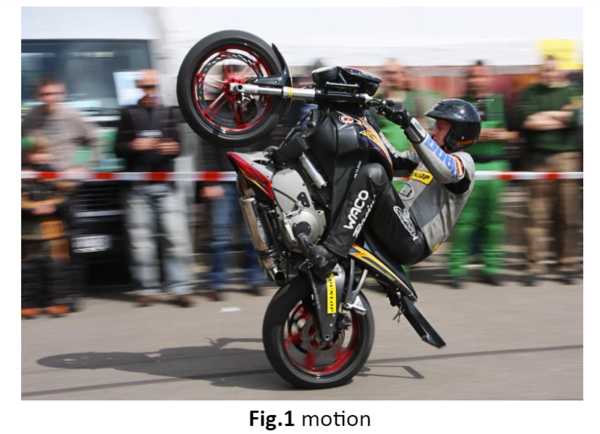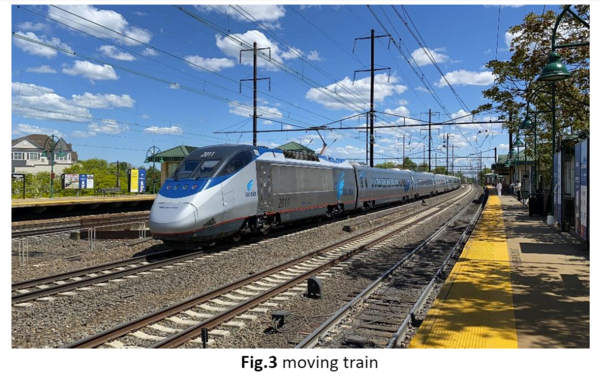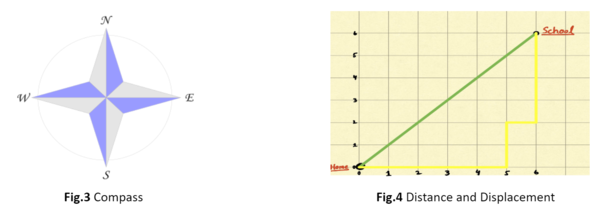• ### Browse All Lessons

##### Assign Lesson

Help Teaching subscribers can assign lessons to their students to review online!

 Tweet# Motion

This lesson aligns with Next Generation Science Standards (NGSS) PS2.A

Introduction
The building block of matter, that is, the atom is in constant motion. A brick is falling from a building, rattling windows, water is flowing out from the pipeline, etc., all exhibit motion. Even the air you breathe exhibits motion. An object is said to be in motion if its distance from other objects is changing. A motion is a phenomenon in which an object or body changes its position regarding time. To describe the state of motion of an object, it must be compared with a fixed point known as a reference point. In this article, we will learn about motion, reference points, distance, and displacement.

Motion
Motion can be defined as any change in the position of a body or an object with respect to its surroundings in a given interval of time. Examples include are; a ball rolling around, a fan, a moving bike, etc.  Everything in the universe is in motion of some kind. The motion can be fast or slow, but it exists. Motion is mainly described in terms of distance, displacement, speed, and time. To decide the state of motion, it is compared with a reference point.Reference point
A fixed point with respect to which an object changes its position is known as a reference point. Stationary objects make a good reference point. For example, Mike is traveling on a train, and when he looks outside from the window, the objects such as buildings, trees, and flagpoles seem to move past the train. Mike can use these objects as a reference point. From this point of view of Mike, these objects are not in motion. It is Mike and the train that is moving with respect to buildings, trees, etc.Distance and Displacement
The two quantities that can be used to describe the change in position are; distance and displacement. Both are discussed below.

Distance
Distance refers to the total path length covered during the motion. It requires only magnitude for its representation. Thus, distance is the total movement of a body without considering its starting and ending points. It is a scalar quantity and can have only positive values.

The distance can also be calculated as the sum of multiple segments. If the total distance between two points is broken down into multiple shorter lengths, then the total distance can be obtained by summation of all those lengths.

$d =d_1 + d_2 + d_3 ...$

Example
For instance, in figure 4 below, a girl takes a yellow route to go to school each morning. From the starting position, e.g., home, she walks 5 blocks, turns left, and covers 2 more blocks. Then she turns right and continues for another block. At the intersection, she turns left and walks 4 blocks to reach her final destination, e.g., school. Now, the length of the segments of her walk from home to school is 5 blocks, 2 blocks, 1 block, and 4 blocks. Thus, she walks from home to school in a total distance of 12 blocks.Displacement
Displacement refers to the shortest distance between the starting and ending positions. It is a vector quantity. It requires not only magnitude but direction as well for its complete representation. An arrow is used to represent the displacement quantity that points from the initial position to the final position. Because it is a vector quantity, it can have positive, negative, or zero values.

For displacement, it is important to take into consideration the starting and ending points of an object. Now look at the green route in the above figure, it shows the displacement from starting point. According to figure 4, the displacement of a girl was 6 blocks northeast.

Scalar and Vector
Scalar is a quantity that can be described only by magnitude. Examples of scalar quantities are mass, electric charge, length, etc.

A vector is a quantity that requires both magnitude and direction for its representation. For example, velocity, momentum, and force are vector quantities.

Summary
• Motion refers to the change in the position of an object with respect to time. Every physical process in the universe is an example of motion.
• Distance and displacement are the quantities that are used to describe the change in position.
• Distance is the total path length covered between two points, while the shortest distance between starting and ending points is known as displacement.
• Distance is a scalar quantity, while displacement is a vector quantity.
• Scalar quantities have only magnitude.
• Vector quantities require both magnitude and direction for their representation.

Related Worksheets: